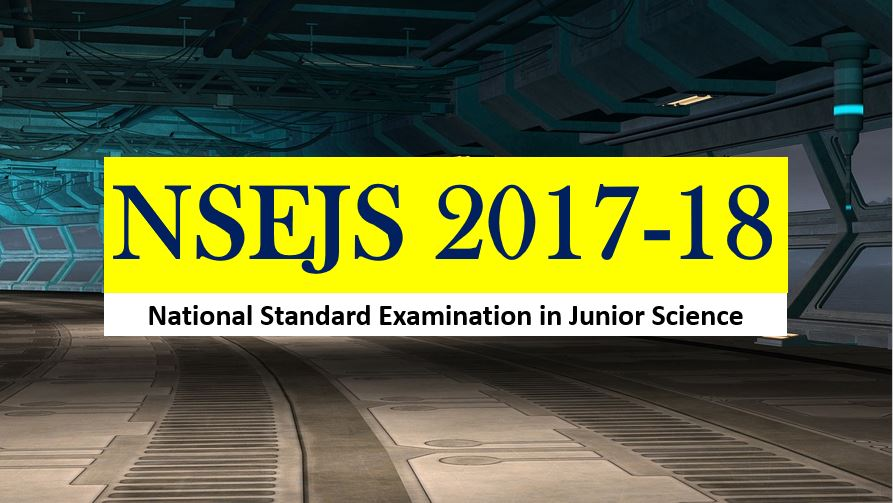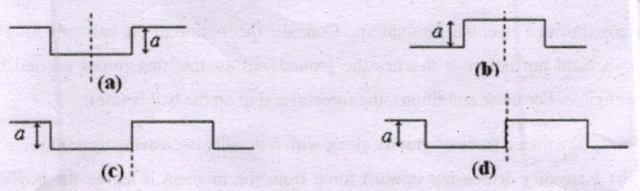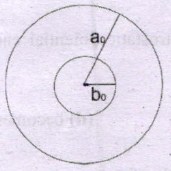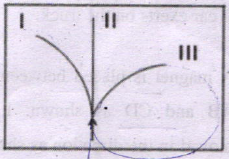Monday, September 27, 2021
Home > Latest Announcement > NSEJS 2017-18 Question Paper With Answer Keys And Solutions

# NSEJS 2017-18 Question Paper With Answer Keys And Solutions# NSEJS 2017-18 Question Paper With Answer Keys And Solutions

Hi students, welcome to AmansMathsBlogs (AMB). On this article, you will get NSEJS 2017-18 Question Paper With Answer Keys And Solutions.

NSEJS 2017-18 is an IJSO Stage 1 exam that is conducted by IAPT (Indian Association of Physics Teacher). In 2017-18 session, NSEJS is conducted on 19 November 2017 (Sunday).

## NSEJS Maths Questions Bank EBook

Get NSEJS 2008 to 2018 Maths Questions Bank with Solutions. To get this combo ebook, click below RED button.NSEJS
Previous Year Question Papers
2008-092009-102010-112011-122012-13
2013-142014-152015-162016-172017-18
2018-192019-20

Get File: Free PDF of 129 Maths Short Tricks (Click On Image)NSEJS 2017-18 Question Paper: Ques No 1

Two wave pulses I and II have the same wavelength. They are travelling in the directions as shown by the single headed arrows. The resultant sketch of the two wave pulses at some instant of time when P coincides with R isOptions:NSEJS 2017-18 Question Paper: Ques No 2

The equivalent resistance of two resistances in series is ‘S’. These resistances are now joined in
parallel. The parallel equivalent resistances is ‘P’. If S = nP. Then the minimum possible value of n is

Options:

A. 2

B. 4

C. 3

D. 5

NSEJS 2017-18 Question Paper: Ques No 3

A copper disc of radius a.has a hole of radius b, at the centre, at 0’C. The disc is now heated and maintained at 200’C. The new radii of disc and hole are a, and b, respectively. For the heated disc it can be concluded that:Options:

A. a0 < at, b0 > bt, and density of disc increases

B. a0 < at, b0 > bt, and density of disc decreases

C. a0 < at, b0 < bt, and density of disc increases

D. a0 < at, b0 < bt, and density of disc decreases

NSEJS 2017-18 Question Paper: Ques No 4

A liquid, whose density doesn’t change during the motion, is flowing steadily through a pipe of varying cross sectional area as shown in the adjacent figure. If a1, a2 are the cross sectional areas, v1, v2 are the values of velocities (or speeds) at L and H respectively, then the correct relation between a1, a2, and v1, v2 is:

Options:

A. a1v1 = a2v2

B. a1v2 = a2v1

C. a12v2 = a22v1

D. a1v12 = a2v22

NSEJS 2017-18 Question Paper: Ques No 5

A common hydrometer has a uniform scale and its stem is graduated downwards from 0 to 20. While floating in water, it reads 0 and while floating in a liquid of density 1.40 g/cm3, it reads 20. Then the density of the liquid in which it will read 10 is ____

Options:

A. 0.7 g/cm3

B. 0.85 g/cm3

C. 1.17 g/cm3

D. 2.8 g/cm3

NSEJS 2017-18 Question Paper: Ques No 6

A boy throws h steel ball straight up. Consider the motion of the ball only after it has left the boy’s hand but before it touches the ground and assume that forces exerted by the air are negligible. For these conditions, the force(s) acting on the ball is (are):

Options:

A. a downward force of gravity along with a steadily decreasing upward force

B. a steadily decreasing upward force from the moment it leaves the boy’s hand until it reaches its highest point; on the way down there is a steadily increasing downward force of gravity as the object gets closer to the earth.

C. constant downward force of gravity along with an upward force that steadily decreases until the ball reaches its highest point; on the way down there is only a constant downward force of gravity

D. constant downward force of gravity only.

NSEJS 2017-18 Question Paper: Ques No 7

In bringing “an α-particle towards another α-particle, the electrostatic potential energy of the system ____

Options:

A. increases

B. decreases

C. remains unchanged

D. becomes zero

NSEJS 2017-18 Question Paper: Ques No 8

An empty office chair is at rest on a floor. Consider the following forces:

I. A downward force of gravity

II. An upward force exerted by the floor,

III. A net downward force exerted by the air

Then, which of the force(s) is (are) acting on the office chair?

Options:

A. I only

B. I and II

C. I, II and III

D. None of Forces

NSEJS 2017-18 Question Paper: Ques No 9

If x, v and t represent displacement (m), velocity (m/s) and time (s) respectively for a certain particle then which pair of the following figures can be best correlated to each other:Options:

A. I and II

B. I and III

C. I and IV

D. None

NSEJS 2017-18 Question Paper: Ques No 10

In rural areas, an indigenous way of keeping kitchen materials cool is to put them in a box and wrap the box with wet blanket; the blanket is kept wet as tap is allowed to drip in to its corner. Choose the correct statement:

Options:

A. This method works because the water from the tap is cold. If one uses room temperature water, it will not work.

B. Method will work only if the box is a bad conductor of heat. If one uses tin box. it will not work.

C. Method doesn’t work.

D. This method works because the latent heat necessary for evaporation of water in the blanket is taken from the box so the box and its content remain cool

NSEJS 2017-18 Question Paper: Ques No 11

An electron and a-particle enter a region of uniform magnetic field (of induction B) with. equal velocities. The direction of B is perpendicular and into the plane of the paper. Then qualitatively identify the direction of path of electron and the path of α-particleOptions:

A. I for α-article and III for electron

B. I for electron and II for α-article

C. I for α-article and II for electron

D. I for electron and III for α-article

NSEJS 2017-18 Question Paper: Ques No 12

A concave mirror of radius of curvature 1 m is placed at the bottom of a water tank. The mirror forms an image of the sun when it is directly overhead. If the depth of water in the tank is 80 cm, then the distance of the image formed is _____ (refractive index of water is 1.33)

Options:

A. 50 cm above mirror

B. on surface of water

C. 110 cm above mirror

D. image cannot be formed

NSEJS 2017-18 Question Paper: Ques No 13

As shown in adjacent figure, two plane mirrors M1 and M2 are inclined to each other at an angle 700 (angle M1OM2). Incident ray AB makes an angle of incidence d on Ml. This ray after reflection at B on M1 and further at C on M2 travels along the direction CD. such that path CD is parallel to M1. Then angle θ isOptions:

A. 45 degree

B. 50 degree

C. 55 degree

D. 60 degree

NSEJS
Previous Year Question Papers
2008-092009-102010-112011-122012-13
2013-142014-152015-162016-172017-18
2018-192019-20

## NSEJS Maths Questions Bank EBook

Get NSEJS 2008 to 2018 Maths Questions Bank with Solutions. To get this combo ebook, click below RED button.Get Online Maths Tuition by AMBiPi for Class 8 to 12